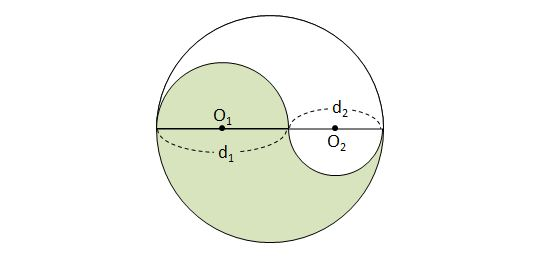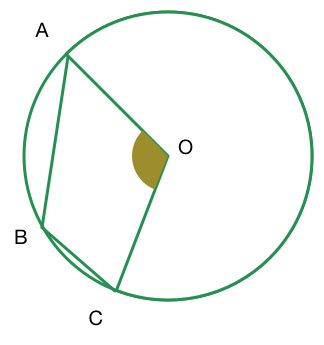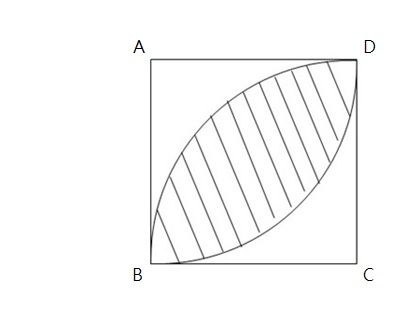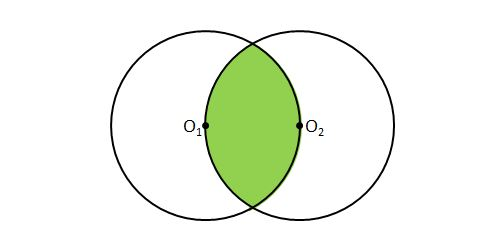Geometry

# Circles Problem SolvingIn the above diagram, $d_1=24$ and $d_2=18.$ What is the perimeter of the green colored region?In the above image of a circle with center at $O,$ $\angle ABC = 115 ^ \circ$. What is angle $AOC$ (as marked) equal to?The length of each side in the above square is $14.$ If the two curves in the square are each one quarter of a circle with radius $14,$ calculate the area of the region bounded by the two curves in the above diagram.

$\Gamma$ is a circle with center $O$. $A$ and $B$ are points on $\Gamma$ such that the sector $AOB$ has perimeter $80$. What is the maximal possible area of sector $AOB$?Let $O_1$ and $O_2$ be the centers of two circles with the same radius $15\text{ cm}.$ What is the perimeter of the green colored region?

×